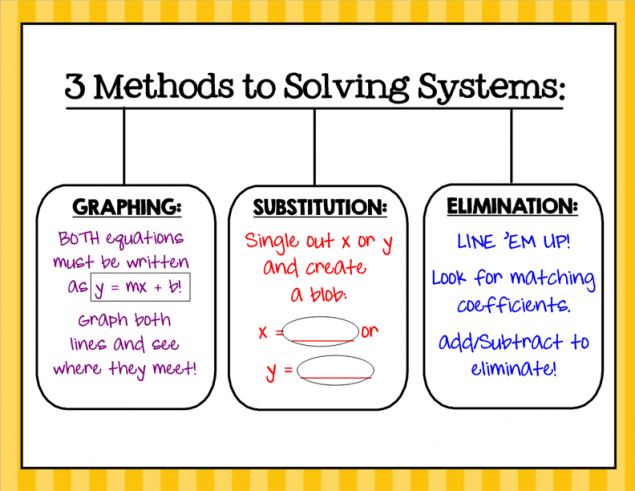# Ms. Minner's Classroom

A Louisville Middle School Classroom

A system of equations includes two or more equations that each involve the same set of two variables. In this module, we will study systems of equations involving two linear equations containing the variables (unknowns) x and y. The solution to a system of equations will be the values that satisfy both equations. These values are written as an ordered pair or point. When graphed, the solution to the system is represented by the point of intersection. Systems of equations can be solved graphically or algebraically. In this module, we will learn how to solve systems of equations by graphing, by substitution, and by elimination.Like equations, systems of equations can have special solutions. A system of linear equations that both have the same slope will have no solutions. They are parallel lines and never intersect. Lines that are identical will overlap and will have infinite solutions. Lines that intersect at one point have one solution. Systems of equations can have one solution, no solutions, or infinite solutions.Systems of Equations Hoop Shoot

Solve Systems by Graphing Practice

Learn Zillion Video

Shmoop Video

Systems Calculator

Solving Systems Calculator

Solving Systems Practice

Jeopardy